# Cremona group

(diff) ← Older revision | Latest revision (diff) | Newer revision → (diff)

The group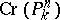of birational automorphisms of a projective space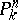over a field; or, equivalently, the group of Cremona transformations (cf. Cremona transformation) of.

The group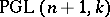of projective transformations ofis contained in a natural manner inas a subgroup; whenit is a proper subgroup. The groupis isomorphic to the group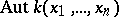of automorphisms overof the field of rational functions invariables over. The fundamental result concerning the Cremona group for the projective plane is Noether's theorem: The group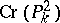over an algebraically closed field is generated by the quadratic transformations or, equivalently, by the standard quadratic transformation and the projective transformations (see , ); relations between these generators can be found in

(see also ). It is not known to date (1987) whether the Cremona group is simple. There is a generalization of Noether's theorem to the case in which the ground fieldis not algebraically closed (see ).

One of the most difficult problems in birational geometry is that of describing the structure of the group, which is no longer generated by the quadratic transformations. Almost all literature on Cremona transformations of three-dimensional space is devoted to concrete examples of such transformations. Finally, practically nothing is known about the structure of the Cremona group for spaces of dimension higher than 3.

An important direction of research in the theory of Cremona groups is the investigation of subgroups of. The finite subgroups of, withalgebraically closed, have been described up to conjugacy (see , and also ). A classification of all involutions inwas obtained as far back as 1877 by E. Bertini (see e.g. , ). The question of describing all involutions in,, remains open. All maximal connected algebraic subgroups inwere described by F. Enriques in 1893 (see ): They are just the automorphism groups of all minimal models of rational surfaces, i.e. of the plane, the quadricand of the series of ruled surfaces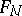,. There are a few generalizations of this result (see , ) to the case of the group,.

How to Cite This Entry:
Cremona group. Encyclopedia of Mathematics. URL: http://encyclopediaofmath.org/index.php?title=Cremona_group&oldid=17476
This article was adapted from an original article by V.A. Iskovskikh (originator), which appeared in Encyclopedia of Mathematics - ISBN 1402006098. See original article